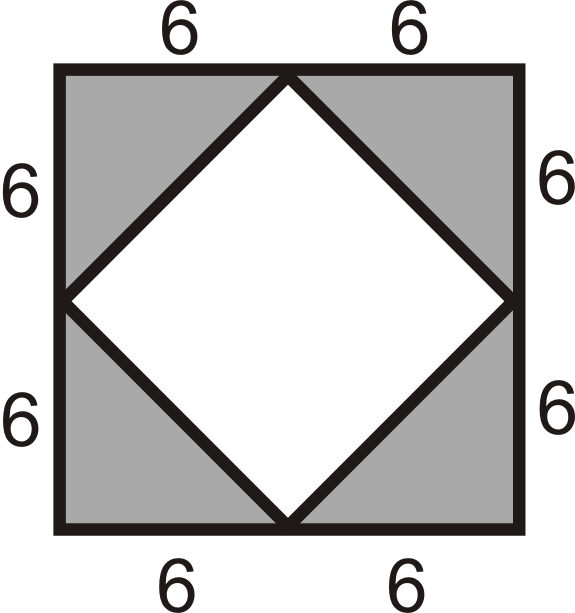# 5.18: Area and Perimeter of Composite Shapes

•• Contributed by CK12
• CK12

Find area and perimeter of figures made up of two or more common shapes.

## Area of Composite Shapes

Perimeter is the distance around a shape. The perimeter of any figure must have a unit of measurement attached to it. If no specific units are given (feet, inches, centimeters, etc.), write “units.”

Area is the amount of space inside a figure. If two figures are congruent, they have the same area (Congruent Areas Postulate).Figure $$\PageIndex{1}$$

A composite shape is a shape made up of other shapes. To find the area of such a shape, simply find the area of each part and add them up.

Area Addition Postulate: If a figure is composed of two or more parts that do not overlap each other, then the area of the figure is the sum of the areas of the parts.

Consider a basic house drawn as a triangle on top of a square. How could you find the area of this composite shape?

Example $$\PageIndex{1}$$

Find the area of the figure below. All angles that look like right angles are right angles.Figure $$\PageIndex{2}$$

Solution

Divide the figure into a triangle and a rectangle with a small rectangle cut out of the lower right-hand corner.Figure $$\PageIndex{3}$$

$$A=A_{\text{top triangle}}+A_{\text{rectangle}}−A_{\text{small triangle}} \\ A=(\dfrac{1}{2} \cdot 6\cdot 9)+(9\cdot 15))−(\dfrac{1}{2}\cdot 3\cdot 6) \\ A&=27+135−9 \\ A&=153\text{ units}^2$$

Example $$\PageIndex{2}$$

Divide the shape into two rectangles and one triangle. Find the area of the two rectangles and triangle. All angles that look like right angles are right angles.Figure $$\PageIndex{4}$$

Solution

Rectangle #1: $$\text{Area} =24(9+12)=504\text{ units}^2$$

Rectangle #2: $$\text{Area}=15(9+12)=315\text{ units}^2$$

Triangle: $$\text{Area} =15(9)2=67.5\text{ units}^2$$

Example $$\PageIndex{3}$$

Find the area of the entire shape from Example 2 (you will need to subtract the area of the small triangle in the lower right-hand corner).

Solution

$$\text{Total Area} =504+315+67.5−\dfrac{15(12)}{2}=796.5 \text{ units}^2$$

Example $$\PageIndex{4}$$Figure $$\PageIndex{5}$$

All angles that look like right angles are right angles.

1. Divide the shape into two triangles and one rectangle.
2. Find the area of the two triangles and rectangle.
3. Find the area of the entire shape.

Solution

1. One triangle on the top and one on the right, the rest of the shape is a rectangle.
2. The area of the triangle on top is $$\dfrac{8(5)}{2}=20\text{ units}^2$$. The area of the triangle on the right is $$\dfrac{5(5)}{2}=12.5\text{ units}^2$$. The area of the rectangle is $$375\text{ units}^2$$.
3. The total area is $$407.5\text{ units}^2$$.

## Review

Use the picture below for questions 1-4. The composite shape is formed of a square within a square.Figure $$\PageIndex{6}$$
1. Find the area of the outer square.
2. Find the area of one grey triangle.
3. Find the area of all four grey triangles.
4. Find the area of the inner square.

Find the areas of the figures below. You may assume all sides are perpendicular.

1.Figure $$\PageIndex{7}$$
2.Figure $$\PageIndex{8}$$

Find the areas of the composite figures.

1.Figure $$\PageIndex{9}$$
2.Figure $$\PageIndex{10}$$
3.Figure $$\PageIndex{11}$$
4.Figure $$\PageIndex{12}$$
5.Figure $$\PageIndex{13}$$
6.Figure $$\PageIndex{14}$$

Use the figure to answer the questions.Figure $$\PageIndex{15}$$
1. What is the area of the square?
2. What is the area of the triangle on the left?
3. What is the area of the composite figure?

## Vocabulary

Term Definition
area The amount of space inside a figure. Area is measured in square units.
composite shape A shape made up of other shapes.
perimeter The distance around a shape. The perimeter of any figure must have a unit of measurement attached to it. If no specific units are given (feet, inches, centimeters, etc), write units.
Composite A number that has more than two factors.